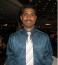# EVM CalculationHi All,

Qus:1 Your project has experienced some changes to the agreed-upon WBS elements. The changes were approved through the proper change control process. The WBS changes might in turn require which of the following?

A.    Scope changes

B.    Cost changes

C.    Schedule revisions

D.    Risk response changes

Correct Answer: C     (I have chosen A)

Qus2: You know that BAC = 500, PV = 325, cumulative AC = 275, and cumulative EV = 250 and that you are experiencing typical variances. What is ETC?

A.    227.3

B.    250

C.    274.7

D.    525

Correct Answer: C  ( What is the correct Formula to be used?)

Regards,

Mani

### I think Kim's

Qi

I think Kim's comprhension is that , because any change in wbs or wp , revision will take place to the base lines.

Here the term scope change or cost change does not mean that it is a base line change, its meaning is like complete change.

And hence only the option C has a term "revision" is appropriate here.

Q2

its a general q , of pmp pattern.

if your concept and practice is ok then its not a question.

EAC = BAC/CPI  [TYPICAL]

CPI = 250/275 =10/11

EAC = 500/10/11 = 550

ETC = EAC- AC = 550-275 = 275~ 274.7### EVM Calculation

Thanks for clarifying it.

Q2: Kim has given a below formula for EVM and i could not interpret with my book (Rita 7th Ed).

ETC =  (BAC - cumulative EV) / cumulative CPI

Q1: I now got clarified with WBS changes. Thanks.### My interpretation of Q1 is

My interpretation of Q1 is that following WBS changes, the next thing to revise would be the schedule, hence C would be correct. Scope change already resulted in WBS changes